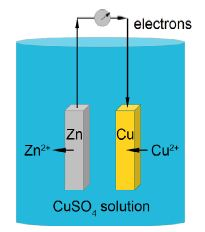Chemistry

# Explain Nernst Equation

The following redox chemical reaction

Zn(s) + Cu2+ → Zn2+ +Cu(s)

can be made into an electrochemical cell to derive electrical energy, below Figure.The cell is called the Daniell cell and was the power behind the telegraph systems in 1830s-1860s that connected up the world in the first high-speed communications system, a forerunner of the internet using the binary code (Morse code). The cell has a voltage difference between the zinc and copper metal electrodes which is given by the Nernst equation

E = E0 – (RT/nF) ln [Zn2+/ Cu2+]

Where E is the voltage under a given set of conditions, E0 is the standard voltage when all the concentrations are 1 mol L-1 (note we ignore the concentrations of the solid electrodes as they are constant), n is the number of electrons transferred in the reaction and in this case n = 2, R is the gas constant, T the temperature in kelvins, and F is a constant called the Faraday constant.

Calculate the concentration of [Zn2+] for E = 1.21 V, [Cu2+] = 0.1 mol L-1, T = 298 K, n = 2, R = 8.314 J K-1 mol L-1, F = 96485 J V-1 = 96485 C mol L-1 where C is a coulomb. and E0 = 1.10 V.

E = E0 – (RT/nF) ln [Zn2+/ Cu2+]

The Nernst equation for the Zn2+/ Cu2+  redox reaction is solved for Zn2+ by: (1) substituting in the known variables; (2) rearranging the equation so that the term containing Zn2+ is the subject; (3) the exponential being evaluated as “shift-In” on most calculators.As well as power sources such as batteries and fuel cells, electrochemical methods are commonly used in analytical chemistry for determining the concentrations of metal ions in drinking water, rivers, lakes, off-shore water, blood, drinks, food products, and in environmental pollution monitoring. Electrochemistry is also used in the industrial production of many important metals e.g. aluminium and magnesium.